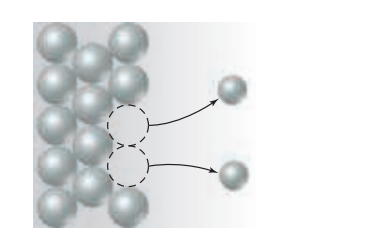×
Get Full Access to Chemistry: The Central Science - 14 Edition - Chapter 20 - Problem 20.3
Get Full Access to Chemistry: The Central Science - 14 Edition - Chapter 20 - Problem 20.3

×ISBN: 9780134414232 1274

## Solution for problem 20.3 Chapter 20

Chemistry: The Central Science | 14th Edition

• Textbook Solutions
• 2901 Step-by-step solutions solved by professors and subject experts
• Get 24/7 help from StudySoup virtual teaching assistantsChemistry: The Central Science | 14th Edition

4 5 1 416 Reviews
13
5
Problem 20.3

The diagram that follows represents a molecular view of a process occurring at an electrode in a voltaic cell.(a) Does the process represent oxidation or reduction?

(b) Is the electrode the anode or cathode?

(c) Why are the atoms in the electrode represented by larger spheres than those in the solution? [Section 20.3]

Step-by-Step Solution:
Step 1 of 3

Ch. 10 The Shapes of Molecules 10.1 Depicting Molecules and Ions with Lewis Structures lewis structure: shows electron-dot symbols for the atoms, the bonding pairs as lines, and lone pairs as dots Applying the Octet Rule to Write Lewis Structures Steps for writing a lewis structure 1. Place the atoms relative to each other, generally the atom with the lower group number in the center, if the atoms are in the same group, place the atom with the higher period number in the center 2. Determine the total number of valence electrons, add up all of the separate valence electrons of the atoms 3. Draw a single bond from each surrounding atom to the central atom, each single bond represents 2 electrons, so subtract the number of single bonds multiplied by two from the total number of valence electrons 4. Distribute the remaining electrons in pairs on the surrounding atoms so that each atom satisfies the octet rule, if any still remain place them around the central atom 5. If you are writing the lewis structure for a polyatomic ion place brackets around the structure and denote the charge 6. If the central atom does not end up with a full octet, change a lone pair on a surrounding atom into another bonding pair to the central atom, forming a double or triple bond Resonance: Delocalized Electron-Pair Bonding - for molecules with double bonds next to single bonds it is often possible to write more than one lewis structure - if a molecule can be written with more than one lewis structure, then neither lewis structure can depict it accurately

Step 2 of 3

Step 3 of 3

##### ISBN: 9780134414232

This textbook survival guide was created for the textbook: Chemistry: The Central Science, edition: 14. Chemistry: The Central Science was written by and is associated to the ISBN: 9780134414232. The full step-by-step solution to problem: 20.3 from chapter: 20 was answered by , our top Chemistry solution expert on 10/03/18, 06:29PM. The answer to “?The diagram that follows represents a molecular view of a process occurring at an electrode in a voltaic cell. (a) Does the process represent oxidation or reduction? (b) Is the electrode the anode or cathode? (c) Why are the atoms in the electrode represented by larger spheres than those in the solution? [Section 20.3]” is broken down into a number of easy to follow steps, and 54 words. Since the solution to 20.3 from 20 chapter was answered, more than 210 students have viewed the full step-by-step answer. This full solution covers the following key subjects: . This expansive textbook survival guide covers 29 chapters, and 2820 solutions.

## Discover and learn what students are asking

Chemistry: The Central Science : Basic Concepts of Chemical Bonding
?Write the Lewis symbol for atoms of each of the following elements: (a) Al, (b) Br, (c) Ar, (d) Sr.

Unlock Textbook Solution